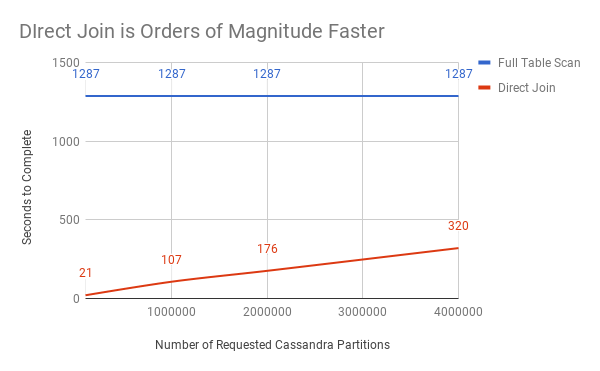# Russell Spitzer's Blog

Some guy's blog

In DSE 6.0 we bring an exciting new optimization to Apache Spark’s Catalyst engine. Previously when doing a Join against a Cassandra Table catalyst would be forced in all cases to perform a full table scan. In some cases this can be extremely inefficient when compared to doing point lookups against a Cassandra table. In the RDD API we added a function `joinWithCassandraTable` which allows doing this optimized join but prior to DSE 6.0 there was no ability to use this in Catalyst. Now in 6.0 a `joinWithCassandraTable` is performed automatically in SparkSQL and DataFrames.

## How to use DSE Direct Join

Lets take a standard join with a Table in DSE with 10 million values in the following schema

``````CREATE TABLE ks.test (
k int PRIMARY KEY,
v int
)
``````

and would like to pull out a small set of keys defined in a CSV. To do this we would use the DataFrame API and perform the following code.

``````val keys = spark
.option("inferSchema", true)
.csv("keys.csv")

val cassandraTable = spark
.cassandraFormat("test", "ks")

keys.join(cassandraTable, keys("k") === cassandraTable("k")).explain
/**
== Physical Plan ==
DSE Direct Join [k = k#77] ks.test - Reading (k, v) Pushed {}
+- *Project [k#77]
+- *Filter isnotnull(k#77)
+- *FileScan csv [k#77] Batched: false, Format: CSV, Location: InMemoryFileIndex[dsefs://127.0.0.1/keys.csv], PartitionFilters: [], PushedFilters: [IsNotNull(k)], ReadSchema: struct<k:int>
**/
``````

In OSS Spark this would cause a full table scan and shuffle inorder to perform the Join, but in DSE 6.0 we avoid both the Full Table Scan and the Shuffle (or BroadcastJoin).

As a user we didn’t have to tell Spark anything about Cassandra or this join, and it automatically made the correct decision.

## Performance

The following is from a RF=3 10 node cluster, 16 cores and 60GB of ram. The total Data set is 10B rows in 20 Million Cassandra Partitions.In this chart we can see that unlike the Full Table Scan, the direct join can efficiently retrieve small amounts of Cassandra Data. This does not even take into account the amount of time additionally required to shuffle the data and actually perform the join in the non-direct join scenario.

Under the hood the direct join is using the joinWithCassandraTable function to do asynchronous point requests. This path also circumvents the normal Spark Connector type conversion allowing it to get rows into Catalyst even faster than joinWithCassandraTable could do otherwise.

## When the DSE Direct Join Is automatically applied

A DirectJoin is substituted in for a Spark Join when the following conditions are met

1. At least one side of the join is a CassandraSourceRelation
2. The join condition fully restricts the partition key
3. The size ratio between the data to join and target table is below directJoinSizeRatio

If these conditions are not met the query plan is left to Spark to optimize.

The Ratio is defined as 0.9 by default but can be changed by setting the “directJoinSizeRatio” option in the Spark Session config.

## Manually Setting Direct Joins

Direct joins can be set to always be used (regardless of size ratio) by setting directJoinSetting to “on”. Setting the parameter to “off” will disable using the optimization even if the ratio is favorable. The default “auto” checks the ratio before either applying or skipping the optimization.

Programatically the decisions can be made on a per table basis using the `directJoin` function. This function can be applied to any DataFrame plan with an underlying CassandraSource relation and will mark the relation as to whether it can or cannot be a direct join target.

### Example

``````scala> keys.join(cassandraTable.directJoin(AlwaysOn), keys("k") === cassandraTable("k")) //Direct Join
scala> keys.join(cassandraTable.directJoin(AlwaysOff), keys("k") === cassandraTable("k")) //Spark Join
scala> keys.join(cassandraTable.directJoin(Automatic), keys("k") === cassandraTable("k")) //Uses size ratio to decide
``````

## How Direct Join Effects the query plan

To see exactly what Spark did let’s call explain on the join.

``````scala> keys.join(cassandraTable, keys("k") === cassandraTable("k")).explain
== Physical Plan ==
DSE Direct Join [k = k#77] ks.test - Reading (k, v) Pushed {}
+- *Project [k#77]
+- *Filter isnotnull(k#77)
+- *FileScan csv [k#77] Batched: false, Format: CSV, Location: InMemoryFileIndex[dsefs://127.0.0.1/keys.csv], PartitionFilters: [], PushedFilters: [IsNotNull(k)], ReadSchema: struct<k:int>
``````

To see what would have happened without Direct Join we can manually disable the directJoin on the Cassandra table with the `directJoin` hint function.

``````scala> keys.join(cassandraTable.directJoin(AlwaysOff), keys("k") === cassandraTable("k")).explain
== Physical Plan ==